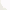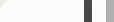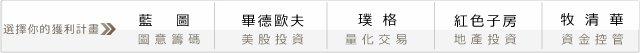## 坊間大絕之CDP逆勢操作系統Ｈ：最高價，L：最低價，O：開盤價，C：收盤價，P：波幅（即最高價減最低價）

(1) 首先求出昨日行情的CDP值：
CDP = ( H + L + 2C ) / 4

(2) 再分別計算昨天行情得最高值(AH)、近高值(NH)、近低值(NL)及最低值(AL)：
AH = CDP + P
NH = 2CDP - L
NL = 2CDP – H
AL = CDP – P

(1) 當指數在近低值附近為買近時機，在近高值附近為賣出時機。（逆勢）

(2) 開盤價即在最高價或最低價附近，代表盤勢強勢十分明顯。

#### 回測時間，SHOW TIME!

input:stoploss(50);
variables:cdp(0),ah(0),nh(0),nl(0),al(0),x(0),y(0);

if date<>date then begin
cdp = (highD(1)+LowD(1)+2*CloseD(1))/4;
ah = cdp + (highD(1) - LowD(1));
nh = cdp*2 - LowD(1);
nl = 2*cdp - highD(1);
al = cdp - (highD(1) - LowD(1));
x = 0;
y = 0;
end;
if time>0850 then begin
if marketposition <=0 and cdp > 0 and x = 0 then begin
Buy next bar at ah stop;
end;
if marketposition >= 0 and cdp > 0 and y = 0 then begin
Sell next bar at al stop;
end;
end;
if marketposition = 1 then begin
x = 1;
end;
if marketposition = -1 then begin
y = 1;
end;
if marketposition = 1 then begin
exitlong next bar at entryprice-stoploss stop;
end;
if marketposition = -1 then begin
exitshort next bar at entryprice + stoploss stop;
end;
if time = 1340 then begin
exitlong on close;
exitshort on close;
cdp = 0;
ah = 0;
nh = 0;
nl = 0;
al = 0;
end;
SetExitOnClose;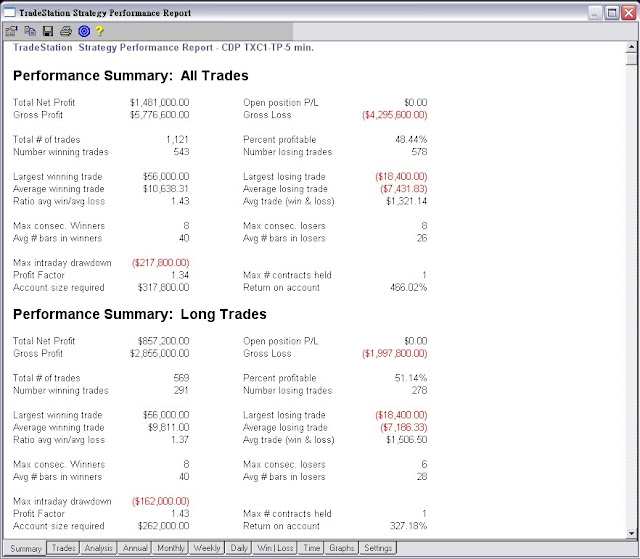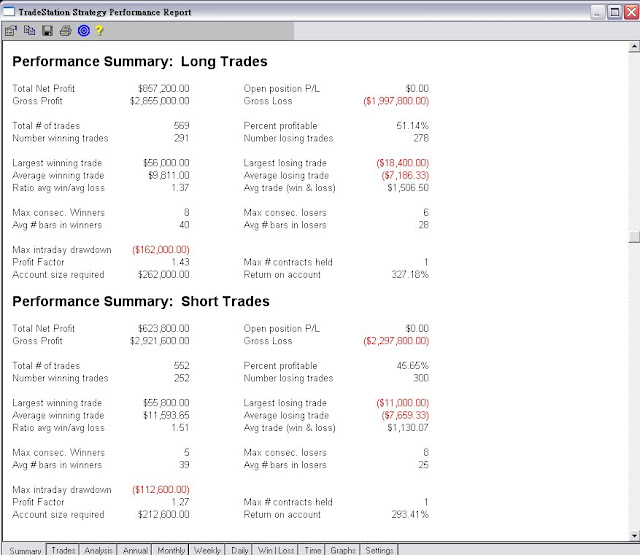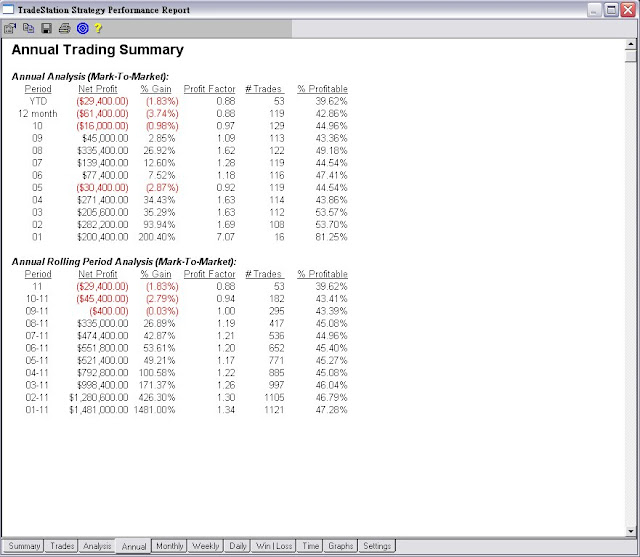2005年、2010年以及今年都是小小賠錢，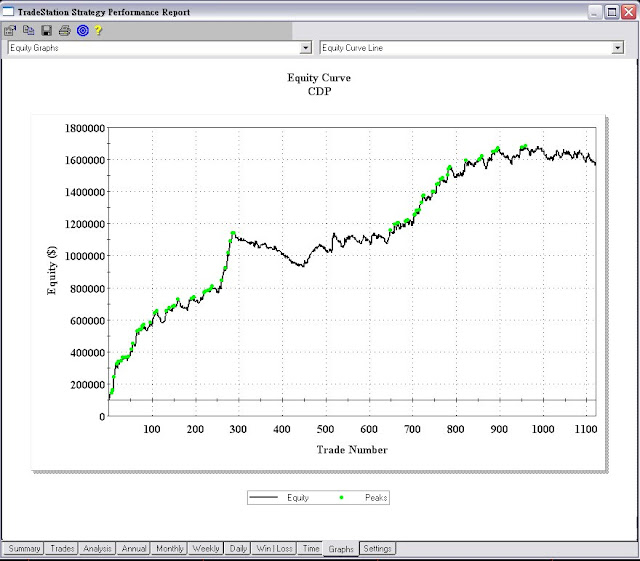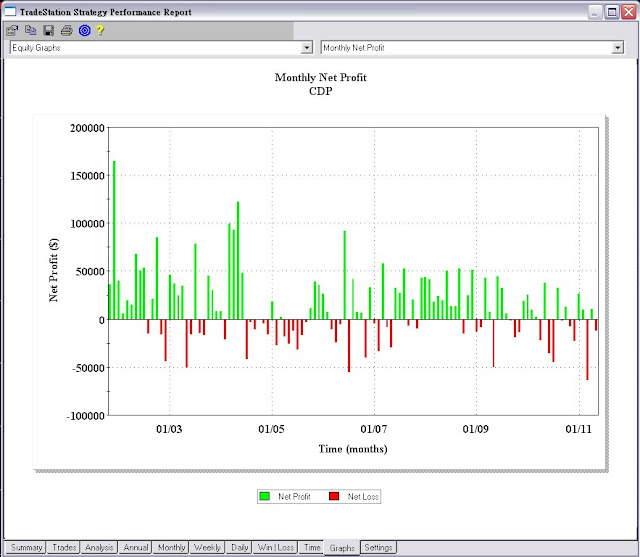input:stoploss(50);
variables:cdp(0),ah(0),nh(0),nl(0),al(0),x(0),y(0),dh(0),dd(99999);
if date<>date then begin
cdp = (highD(1)+LowD(1)+2*CloseD(1))/4;
ah = cdp + (highD(1) - LowD(1));
nh = cdp*2 - LowD(1);
nl = 2*cdp - highD(1);
al = cdp - (highD(1) - LowD(1));
x = 0;
y = 0;
end;
if t=0900 then begin
dh=highd(0);
dd=lowd(0);
end;
if time>0900 then begin
if marketposition <=0 and cdp > 0 and x = 0 and high>dh then begin
Buy next bar at ah stop;
end;
if marketposition >= 0 and cdp > 0 and y =0 and low< dd then begin
Sell next bar at al stop;
end;
end;
if marketposition = 1 then begin
x = 1;
end;
if marketposition = -1 then begin
y = 1;
end;
if marketposition = 1 then begin
exitlong next bar at entryprice-stoploss stop;
end;
if marketposition = -1 then begin
exitshort next bar at entryprice + stoploss stop;
end;
if time = 1340 then begin
exitlong on close;
exitshort on close;
cdp = 0;
ah = 0;
nh = 0;
nl = 0;
al = 0;
end;
SetExitOnClose;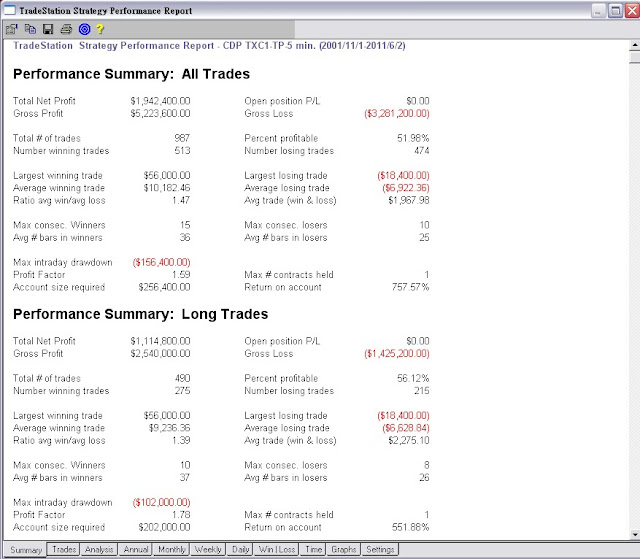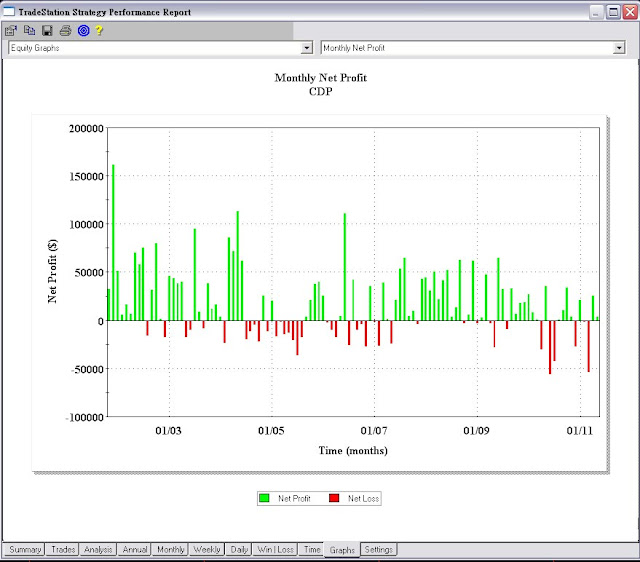#### 0 意見: